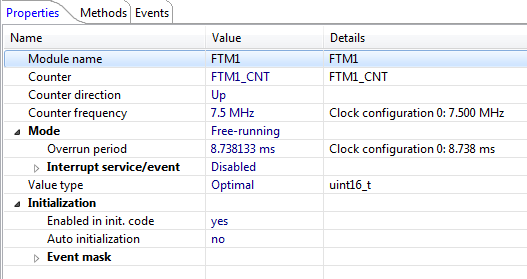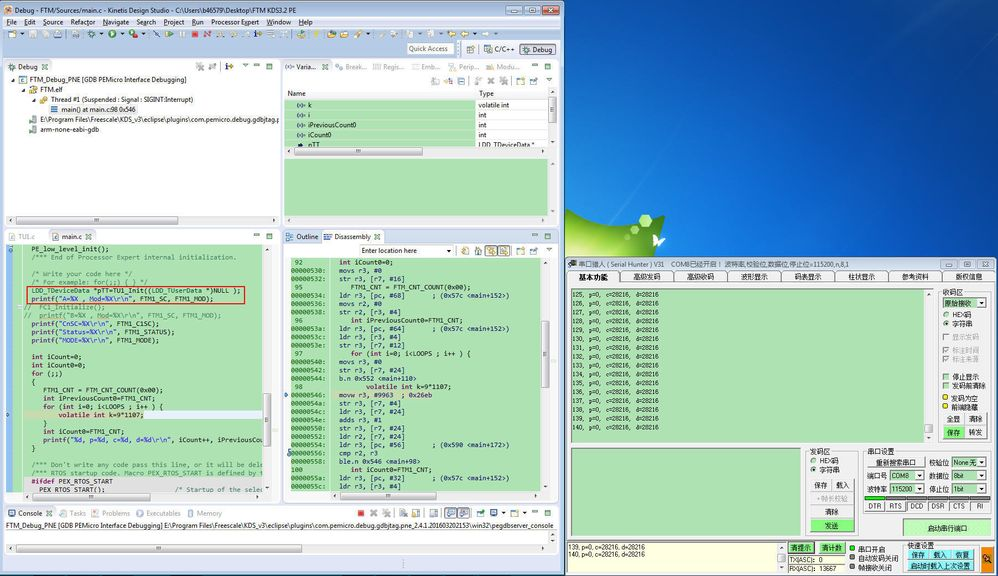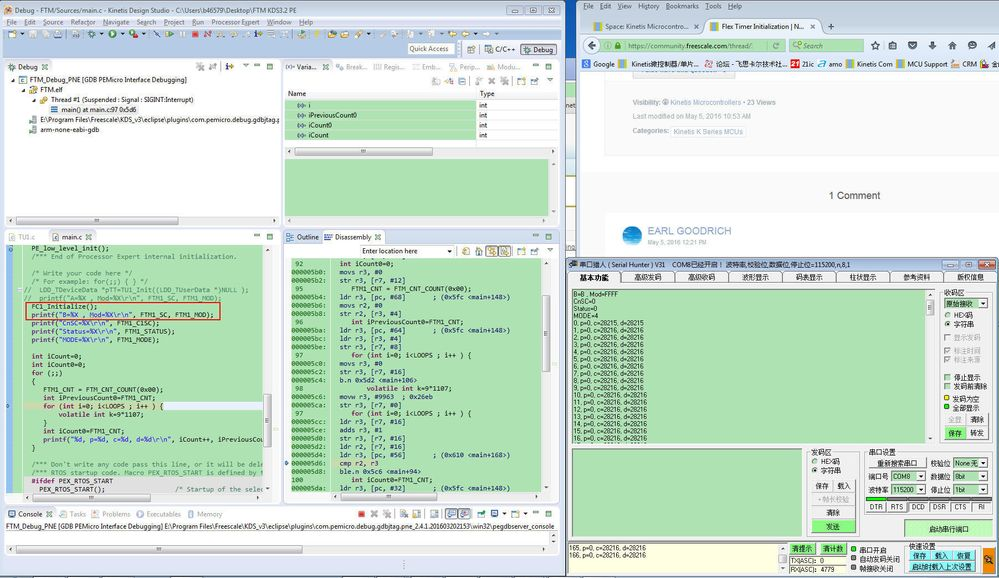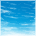## Flex Timer Initialization

cancel
Showing results for
Did you mean:
SOLVED

## Flex Timer Initialization

573 ViewsContributor V

Hi,

I have K60 tower board: K60P144M150SF3RM

I have a dummy question and couldn't figure out. Hope some one can help me give me an answer.

I created a free running counter FTM1_CNT as follows:No interrupt, Counting up with period about 8.738 ms

I run the following code: Basically, Initialize, reset counter value to 0, read, delay some time with loops, and read again. Here is the code:

LDD_TDeviceData *pTT=FC1_Init((LDD_TUserData *)NULL );

printf("A=%X , Mod=%X\r\n", FTM1_SC, FTM1_MOD);

printf("CnSC        =%X\r\n", FTM1_C1SC);

printf("Status    =%X\r\n", FTM1_STATUS);

printf("MODE        =%X\r\n", FTM1_MODE);

int iCount=0;

int iCount0=0;

for (;;)

{

FTM1_CNT = FTM_CNT_COUNT(0x00);

int iPreviousCount0=FTM1_CNT;

for (int i=0; i<LOOPS ; i++ ) {

volatile int k=9*1107;

}

int iCount0=FTM1_CNT;

printf("%d, p=%d, c=%d, d=%d\r\n", iCount++, iPreviousCount0, iCount0, (iCount0 - iPreviousCount0) );

}

Running Results:

A=B , Mod=FFFF

CnSC        =0

Status    =0

MODE        =4

0, p=0, c=28409, d=28409

1, p=0, c=28329, d=28329

2, p=0, c=28384, d=28384

3, p=0, c=28329, d=28329

4, p=0, c=28383, d=28383

5, p=0, c=28326, d=28326

6, p=0, c=28378, d=28378

7, p=0, c=28329, d=28329

8, p=0, c=28382, d=28382

9, p=0, c=28329, d=28329

10, p=0, c=28379, d=28379

But when I initialize the same counter with the following code (They are the almost same as FC1_Init() by PE:)

I copied from PE generated code into my own initialization function:

void FC1_Initialize() {

/* SIM_SCGC6: FTM1=1 */

/* FTM1_MODE: FAULTIE=0,FAULTM=0,CAPTEST=0,PWMSYNC=0,WPDIS=1,INIT=0,FTMEN=0 */

FTM1_MODE = (FTM_MODE_FAULTM(0x00) | FTM_MODE_WPDIS_MASK); /* Set up mode register */

/* FTM1_SC: TOF=0,TOIE=0,CPWMS=0,CLKS=0,PS=0 */

FTM1_SC = (FTM_SC_CLKS(0x00) | FTM_SC_PS(0x00)); /* Clear status and control register */

/* FTM1_CNTIN: INIT=0 */

FTM1_CNTIN = FTM_CNTIN_INIT(0x00);   /* Clear counter initial register */

/* FTM1_CNT:  COUNT=0 */

FTM1_CNT = FTM_CNT_COUNT(0x00);      /* Reset counter register */

/* FTM1_C0SC:  CHF=0,CHIE=0,MSB=0,MSA=0,ELSB=0,ELSA=0,??=0,DMA=0 */

FTM1_C0SC = 0x00U;   /* Clear channel status and control register */

/* FTM1_C1SC: CHF=0,CHIE=0,MSB=0,MSA=0,ELSB=0,ELSA=0,??=0,DMA=0 */

FTM1_C1SC = 0x00U;   /* Clear channel status and control register */

/* FTM1_MOD: MOD=0xFFFF */

FTM1_MOD = FTM_MOD_MOD(0xFFFF);      /* Set up modulo register */

_int_disable();

FTM_PDD_SelectPrescalerSource(FTM1_BASE_PTR, FTM_PDD_DISABLED);

FTM_PDD_SetPrescaler(FTM1_BASE_PTR,  FTM_PDD_DIVIDE_8 ); /* Set prescaler register */

FTM_PDD_SelectPrescalerSource(FTM1_BASE_PTR, FTM_PDD_SYSTEM);

_int_enable();

}

I run the same code as above, except the initialization FC1_Initialize():

{

int iCount=0;

int iCount0=0;

FC1_Initialize();

printf("B=%X , Mod=%X\r\n", FTM1_SC, FTM1_MOD);

printf("CnSC        =%X\r\n", FTM1_C1SC);

printf("Status    =%X\r\n", FTM1_STATUS);

printf("MODE        =%X\r\n", FTM1_MODE);

for (;;)

{

FTM1_CNT = FTM_CNT_COUNT(0x00);

int iPreviousCount0=FTM1_CNT;

for (int i=0; i<LOOPS ; i++ ) {

volatile int k=9*1107;

}

int iCount0=FTM1_CNT;

printf("%d, p=%d, c=%d, d=%d\r\n", iCount++, iPreviousCount0, iCount0, (iCount0 - iPreviousCount0) );

}

The running results:

B=B , Mod=FFFF

CnSC        =0

Status    =0

MODE        =4

0, p=0, c=40915, d=40915

1, p=0, c=40884, d=40884

2, p=0, c=40882, d=40882

3, p=0, c=40831, d=40831

4, p=0, c=40827, d=40827

5, p=0, c=40881, d=40881

6, p=0, c=40882, d=40882

7, p=0, c=40883, d=40883

8, p=0, c=40882, d=40882

9, p=0, c=40881, d=40881

10, p=0, c=40882, d=40882

But the duration for the same loops are huge different:

#define LOOPS  100000

for (int i=0; i<LOOPS ; i++ ) {

volatile int k=9*1107;

}

d=28329  vs d=40915    :: Why?

I don't know what causes that difference.

Both are configured as

clock source: system clock:      (01)

The counter prescaler :  011 (FTM_PDD_DIVIDE_8),

FTM1_SC = 0xB

FTM1_MOD = 0xFFFF

But the counter is running at different rate.

What else is different?

Thank you,

David Zhou

Labels (1)
• ### Kinetis K Series MCUs

1 Solution
151 ViewsNXP TechSupport

Hi David,

I am testing your code in KDS3.2.0 with Processor Expert.

Only modify the Initialize function in one project, and the Optimization Level is NONE.

I think you can check the code in Disassembly.Best Regards,

Robin

4 Replies
152 ViewsNXP TechSupport

Hi David,

I am testing your code in KDS3.2.0 with Processor Expert.

Only modify the Initialize function in one project, and the Optimization Level is NONE.

I think you can check the code in Disassembly.Best Regards,

Robin

151 ViewsContributor V

Earl and Robin,

Thank your for testing the code for me. I still believe something else causing the issue. I created another timer to measure that first timer, and I printed out all the registers. I found that the SC was different. In the PE the divider is set to 8 (011 binary for PS filed) but it changed to 00 with clock source to external instead of system clock.

One thing confused me is that in my previous project, SC are the same (0xB) for both initialization, but runs at different rate.

OK. I am closing this tick. Thank both of you.

David Zhou

151 ViewsSenior Contributor III

And you might note that these particular results show the 'same loops' aligned in FLASH on the SAME Phrase (starting address ends in 0b...1000110) boundaries, so the timing result is the SAME.  YMMV.

151 ViewsSenior Contributor III

Because in a superpipelined architecture with Flash pre-fetch, burst-access, and 'cache', and any NUMBER of other alterations to 'clocks per instruction' you cannot count on any particular 'loop of counts' to take any PARTICULAR time, even between compile/link that is likely to move the boundaries.  Welcome to the world of 'performance CPUs'.

And THAT assumes that the compiler created exactly the same instructions for the two loops, which is a big 'if' given the changes in context.

Check your 'counter rate' by some other means, such as triggering an I/O and watching on a scope.

 GPIOE_PTOR = 1<<17; //Time the loops on E17!